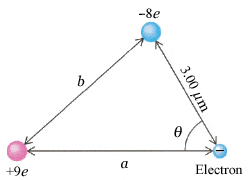# Electric Forces between three charges

thst1003An electron is near a positive ion of charge +9e and a negative ion of charge −8e (see the figure below). (Take a = 5.23 µm, b = 4.28 µm, and θ = 54.9°.)

(a) Find the magnitude and direction of the resultant force on the electron. (Let right be the +x-direction. Measure the angle counter-clockwise from the +x-axis.)

F = k(q x q/r^2)

I tried using this equation and then finally doing vector addition and I got an answer of: 2.235e-16 N and this was wrong.

## Answers and Replies

zhermes
Then I guess you used the equation or did the vector addition wrong....

Care to show some of your work?

thst1003
Thanks! I actually was using my calculator in the incorrect mode. I figure it out. I really appreciate it.